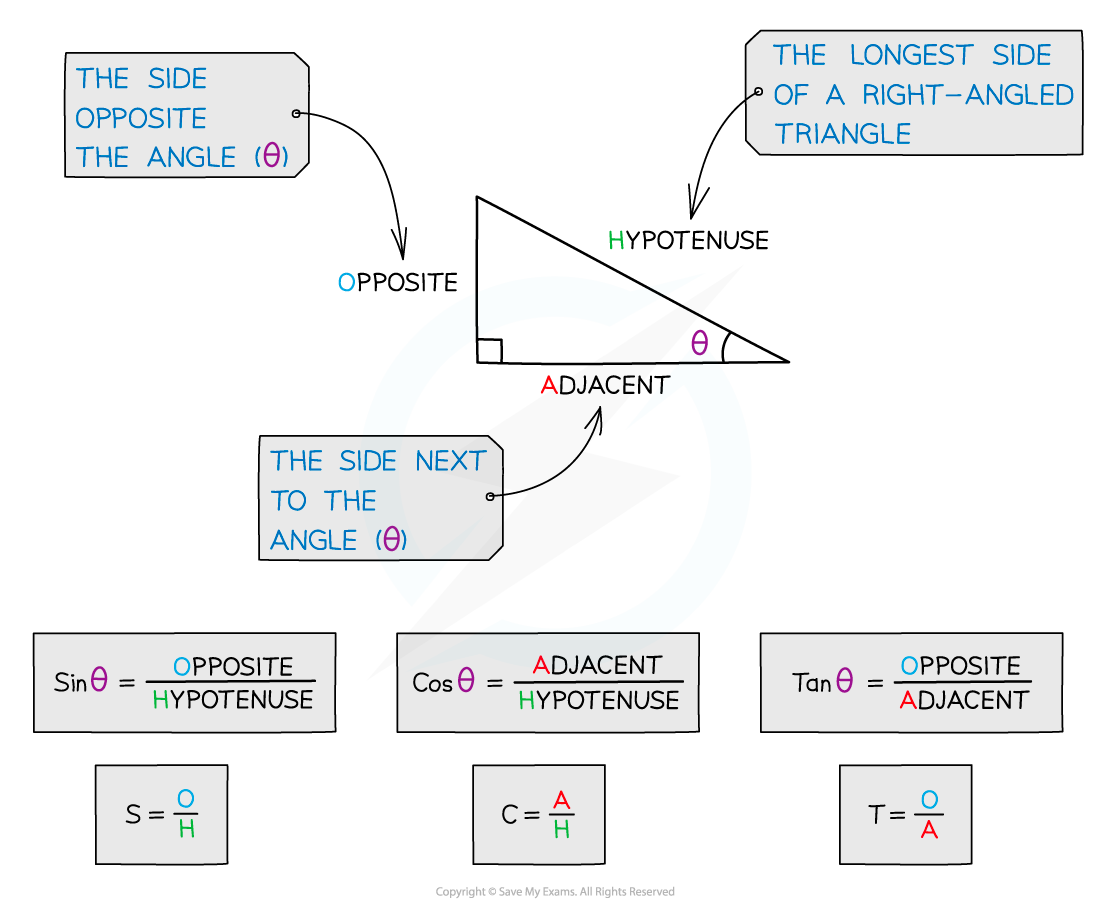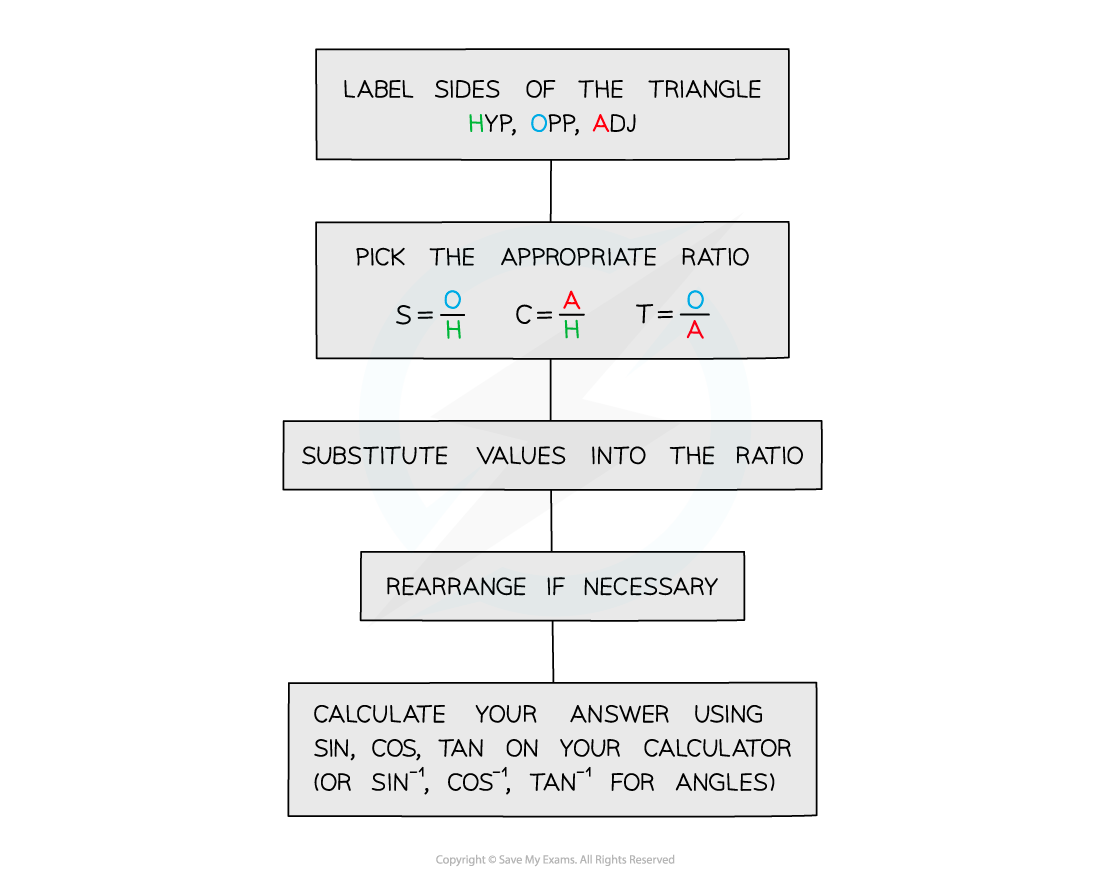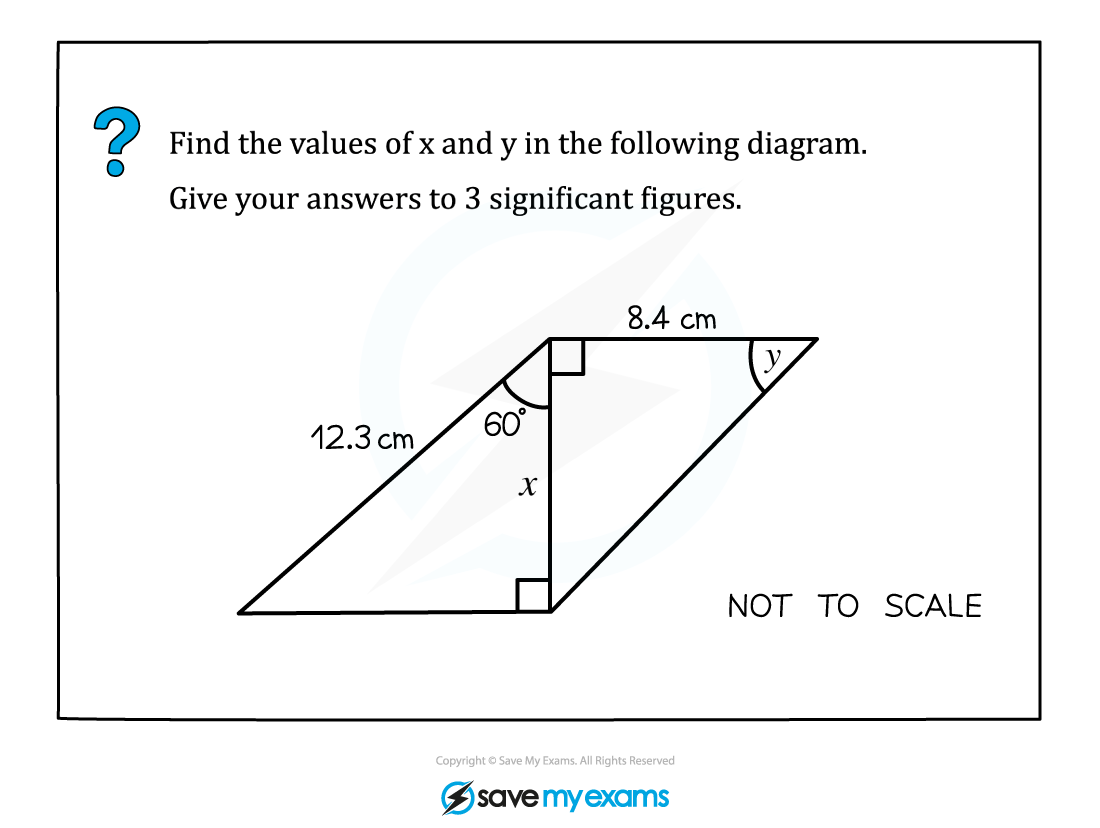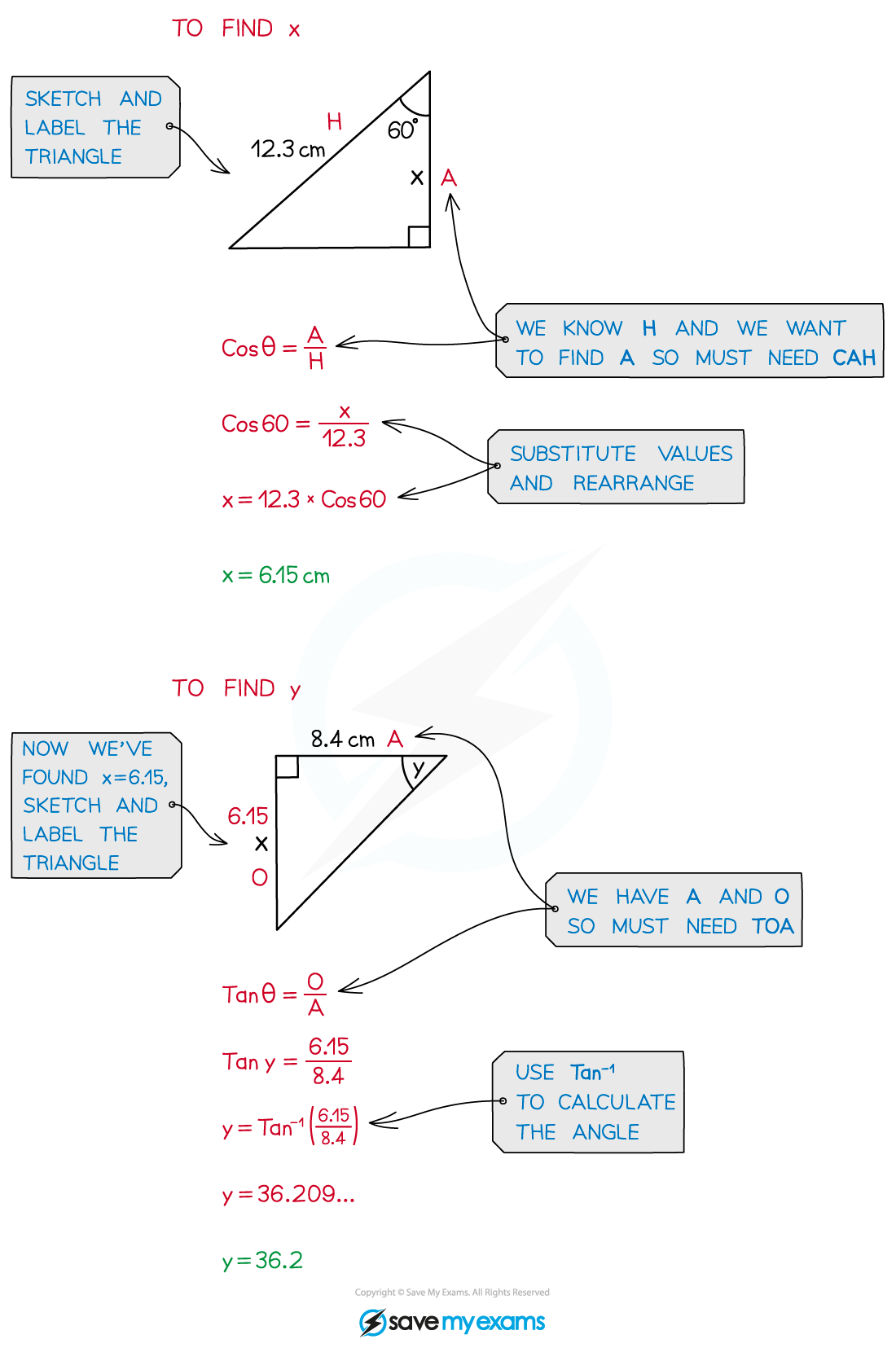# AQA A Level Maths: Pure复习笔记5.1.2 Right-Angled Triangles

### Right-Angled Triangles

#### What is SOHCAHTOA?

• SOH, CAH, and TOA are an easy way to remember the three basic trigonometry ratios
• They show the relationship between an angle and the different sides of a right-angled triangle which we can label Hypotenuse, Adjacent and Opposite
• We use them to find missing angles and sides for right‑angled triangles
• Hypotenuse is the longest side of a right-angled triangle
• Adjacent is the side next to the angle you are working with (in between the angle and the right angle)
• Opposite is the side opposite the angle you are working with•  We use the inverse trig functions to find missing angles#### How do I use SOHCAHTOA?#### Exam Tip

• Remember SOHCAHTOA are only for calculating missing sides and angles for right‑angled triangles
• For non-right‑angled triangles you will need to use the Sine or Cosine rules.
• Make sure your calculator is in degree mode (D).
• Always check your answers are sensible…
• Should my answer be longer or shorter than the sides I have already i.e. is it the hypotenuse?
• Is my angle sensible…
• Have I remembered to use -1?
• Check if the question asks you to round your answer.

#### Worked Example